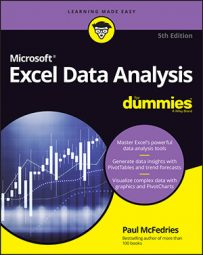##### Excel Data Analysis For DummiesData analysis, by definition, requires some data to analyze. However, after you’ve imported or entered that data and cleaned it up as best you can. what’s your next move? Ah, that’s where the “analysis” part raises its hand in the air and says, “Pick me, pick me!”

Excel is bursting at its digital seams with tools for analyzing data, but there are some that you’ll turn to most often. Here you’ll find quick guides for Excel’s descriptive statistics tools, building comparison expressions, and wielding the powerful database functions.

## Excel's descriptive statistics functions

When it’s time to get down to analyzing your data, a good place to start is with some basic statistics, such as counting items, calculating sums and averages, finding the largest and smallest values, working out the standard deviation, and so on. These measures fall under the general rubric of descriptive statistics, and Excel offers a fistful of functions that help you get the job done. Here’s a summary:

 Function What it does COUNT(value1[, value2, …]) Counts numbers COUNTA(value1[, value2, …]) Counts non-empty cells COUNTBLANK(value1[, value2, …]) Counts empty cells COUNTIF(range, criteria) Counts the cells in a range that match the criteria COUNTIFS(range1, criteria1[, range2, criteria2, …]) Counts the cells in multiple ranges that match multiple criteria PERMUT(number, number_chosen) Counts the permutations COMBIN(number, number_chosen) Counts the combinations SUM(number1[, number2, …]) Calculates the sum SUMIF(range, criteria[, average_range]) Calculates the sum of the cells that match the criteria SUMIFS(average_range, range1, criteria1[, range2, criteria2…]) Calculates the sum of cells in multiple ranges that match multiple criteria AVERAGE(number1[, number2, …]) Calculates the arithmetic mean AVERAGEIF(range, criteria[, average_range]) Calculates the mean of the cells that match the criteria AVERAGEIFS(average_range, range1, criteria1[, range2, criteria2…]) Calculates the mean of cells in multiple ranges that match multiple criteria MEDIAN(number1[, number2, …]) Calculates the median (middle) value MODE(number1[, number2, …]) Calculates the mode (most common) value RANK.EQ(number, ref[, order]) Returns an item’s rank relative to the other items in a data set LARGE(array, k) Returns the kth largest item in a data set SMALL(array, k) Returns the kth smallest item in a data set FREQUENCY(data_array, bins_array) Creates a grouped frequency distribution VAR.S(number1[, number2, …]) Calculates the variance of a sample VAR.P(number1[, number2, …]) Calculates the variance of a population STDEV.S(number1[, number2, …]) Calculates the standard deviation of a sample STDEV.P(number1[, number2, …]) Calculates the standard deviation of a population CORREL(array1, array2) Calculates the correlation between two data sets

## Building comparison expressions

A comparison expression— also known as a logical expression or a Boolean expression — is an expression where you compare the items in a range or table column with a value you specify. In Excel, you use comparison expressions to create advanced filters for a table, as well as in functions that require criteria, such as COUNTIF, SUMIF, and AVERAGEIF.

To construct a comparison expression, you enter a comparison operator from the following table, then a value used in the comparison.

 Operator Name Example What it matches = Equals = 100 Cells that contain the value 100 <> Not equal to <> 0 Cells that contain a value other than 0 > Greater than > 1000 Cells that contain a value greater than 1,000 >= Greater than or equal to >= 25 Cells that contain a value that’s equal to or greater than 25 < Less than < 0 Cells that contain a negative value <= Less than or equal to <= 927 Cells the contain a value that’s equal to or less than 927

## Excel's database functions

To help you analyze data that’s stored in a table or range, you can turn to Excel’s powerful database functions, which enable you to apply calculations such as sum, average, and standard deviation.

The database functions all use the same three arguments:

• Database: The range of cells that make up the table you want to work with. You can use either the table name or the table range address. If you go with the table name, be sure to reference the entire table by using the syntax Table[#All] (where Table is the name of your table).
• Field: A reference to the table column on which you want to perform the operation. You can use either the column header or the column number (where the leftmost column is 1, the next column is 2, and so on). If you use the column name, enclose it in quotation marks (for example, “Unit Price”).
• Criteria: The range of cells that hold the criteria you want to work with. You can use either a range name, if one is defined, or the range address.
 Function What it does DGET(database, field, criteria) Retrieves a value from a table or range DSUM(database, field, criteria) Adds values from a table or range based on the criteria you specify DCOUNT(database, field, criteria) Counts the numeric values in a table or range that match the specified criteria DCOUNTA(database, field, criteria) Counts the non-blank items in a table or range that match the specified criteria DAVERAGE(database, field, criteria) Averages the values in a table or range that match the specified criteria DMAX(database, field, criteria) Finds the maximum value in a table or range based on the criteria you specify DMIN(database, field, criteria) Finds the minimum value in a table or range based on the criteria you specify DPRODUCT(database, field, criteria) Multiplies the values in a table or range that match the specified criteria DSTDEV(database, field, criteria) Calculates the standard deviation of the sample values in a table or range that match the specified criteria DSTDEVP(database, field, criteria) Calculates the standard deviation of the population values in a table or range that match the specified criteria DVAR(database, field, criteria) Calculates the variance of the sample values in a table or range that match the specified criteria DVARP(database, field, criteria) Calculates the variance of the population values in a table or range that match the specified criteria